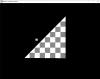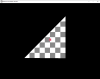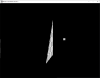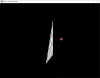# 3D[SOLVED] Point in triangle calculation is very unprecise

#### Kentae

##### Member
Hi, I have a bit of an issue with my point in triangle script.
As the title suggest it is quite unprecise, or rather, it seems to think that the triangle is 10-15 pixels thick.

Here are some example pictures:
The point is represented by the small cube that is only 1 pixel wide/deep/tall. (the cube shape is not part of the calculation. It was just a simple way of visualizing the point)
If you look at the triangle plane head on like in the first two pictures the collision is completley precise.But if you have a look at the collision from the side like in the next two pics you'll see how unprecise the collision is.As you can see in the last picture, the cube that represents the point turns red (indicating that there is a collision) waaay before it hits the triangle plane.

Here's the code:
GML:
``````/// @desc kt_collision_point_tri()

/// @param point_x
/// @param point_y
/// @param point_z
/// @param tri_x1
/// @param tri_y1
/// @param tri_z1
/// @param tri_x2
/// @param tri_y2
/// @param tri_z2
/// @param tri_x3
/// @param tri_y3
/// @param tri_z3

// Get user input.
// Get the coordinates of the point and of the three vetecies that make up the triangle.
var px = argument0;
var py = argument1;
var pz = argument2;
var tx1 = argument3;
var ty1 = argument4;
var tz1 = argument5;
var tx2 = argument6;
var ty2 = argument7;
var tz2 = argument8;
var tx3 = argument9;
var ty3 = argument10;
var tz3 = argument11;

// Subtract the position of the point from the triangle's vertecies.
// We do this so that we can use the origin of the world as the point.
// This just makes the calculation easier.
tx1 -= px;
ty1 -= py;
tz1 -= pz;
tx2 -= px;
ty2 -= py;
tz2 -= pz;
tx3 -= px;
ty3 -= py;
tz3 -= pz;

// Now we are going to pretend that the triangle and the point make up a pyramid.
// We then get the normals of the walls of this pyramid.
var u = cross_product( tx2, ty2, tz2, tx3, ty3, tz3 );
var v = cross_product( tx3, ty3, tz3, tx1, ty1, tz1 );
var w = cross_product( tx1, ty1, tz1, tx2, ty2, tz2 );

// now we check if those normals point in the same direction.
// Return false if they do not.
if ( dot_product_3d( u, u, u, v, v, v ) < 0 )
{
return false;
}
if ( dot_product_3d( u, u, u, w, w, w ) < 0 )
{
return false;
}

// If all normals are pointing in the same direction, return true.
return true;``````
I was following a tutorial I found, so I don't know if I've made a mistake or if this way of finding if a point is in a triangle is inherently unprecise.

Any help would be greatly appreciatedEdit: the cross_product script is one I wrote myself too but that one has been tested propely and works as it should.

#### TheSnidr

##### Heavy metal viking dentist
GMC Elder
Well, the script you've made should return true when the given point's projection onto the plane of the triangle is inside the triangle. For this purpose it seems like it does exactly as it should. If you'd like to know if the point is inside the triangle on screen, you'll need to either transform the 2D screen space point into a 3D vector and do a ray-triangle-intersection algo, or transform the triangle to screens pace and check if the point is inside the 2D triangle.

#### FrostyCat

##### Member
Your code is for point-over-triangle, not for point-in-triangle. You need to add a distance-to-plane check on top of what you have.

#### Kentae

##### Member
All I need is to be able to take any point in 3D space and check if it intersects a given 3D triangle in any way.

Your code is for point-over-triangle, not for point-in-triangle. You need to add a distance-to-plane check on top of what you have.
That makes sence. The tutorial I found said "point in triangle" so that's what I thought it was.

I have, whilst waiting for an answer here, gone through another tutorial that alowed me to check for a sphere intersecting with a triangle.
This script works perfectly and I believe I can transfer the distance-to-plane checking from there.

I guess the title of the original tutorial was a tad missleadingThanks a lot for the help so far @TheSnidr and @FrostyCatI'll do some tests and post here again if I find the solution or if I find more problems hehe ^^'

#### Kentae

##### Member
Allright! I found a solutionI added this to the code:
GML:
``````var pn = cross_product( tx2 - tx1, ty2 - ty1, tz2 - tz1, tx3 - tx1, ty3 - ty1, tz3 - tz1 );
var dis = pn * px + pn * py + pn * pz;

if ( dot_product_3d_normalized( px, py, pz, pn, pn, pn ) - dis != 0 ) return false;``````
This checks for an intersection with the plane of the triangle.

It is however quite difficult to get a collision with triangles that are not axis aligned as the plane has no real "thickness" to it.
With an axis aligned triangle I can just set the point's X, Y or Z to whatever plane the triangle lies on to test it.
So the next step would be to, sort of, add some "thickness" to the plane so that it is easier to get a positive result.
I know that seems like I might try to do the oposite of what I originally asked for help with but i'm looking for a "thickness" of
like 1-2 pixels at most, contra the 10-15 it seemed to have without the plane checking.

Here's the current full code btw, just in case someone else would find it usefullCode:
``````/// @desc kt_collision_point_tri()

/// @param point_x
/// @param point_y
/// @param point_z
/// @param tri_x1
/// @param tri_y1
/// @param tri_z1
/// @param tri_x2
/// @param tri_y2
/// @param tri_z2
/// @param tri_x3
/// @param tri_y3
/// @param tri_z3

// Get user input.
// Get the coordinates of the point and of the three vetecies that make up the triangle.
var px = argument0;
var py = argument1;
var pz = argument2;
var tx1 = argument3;
var ty1 = argument4;
var tz1 = argument5;
var tx2 = argument6;
var ty2 = argument7;
var tz2 = argument8;
var tx3 = argument9;
var ty3 = argument10;
var tz3 = argument11;

// Get the normal vector of the triangle plane.
var pn = cross_product( tx2 - tx1, ty2 - ty1, tz2 - tz1, tx3 - tx1, ty3 - ty1, tz3 - tz1 );
var dis = pn * px + pn * py + pn * pz;

if ( dot_product_3d_normalized( px, py, pz, pn, pn, pn ) - dis != 0 ) return false;

// Subtract the position of the point from the triangle's vertecies.
// We do this so that we can use the origin of the world as the point.
// This just makes the calculation easier.
tx1 -= px;
ty1 -= py;
tz1 -= pz;
tx2 -= px;
ty2 -= py;
tz2 -= pz;
tx3 -= px;
ty3 -= py;
tz3 -= pz;

// Now we are going to pretend that the triangle and the point make up a pyramid.
// We then get the normals of the walls of this pyramid.
var u = cross_product( tx2, ty2, tz2, tx3, ty3, tz3 );
var v = cross_product( tx3, ty3, tz3, tx1, ty1, tz1 );
var w = cross_product( tx1, ty1, tz1, tx2, ty2, tz2 );

// now we check if those normals point in the same direction.
// Return false if they do not.
if ( dot_product_3d( u, u, u, v, v, v ) < 0 )
{
return false;
}
if ( dot_product_3d( u, u, u, w, w, w ) < 0 )
{
return false;
}

// If all normals are pointing in the same direction, return true.
return true;``````